Next: The general LP formulation Up: The LP formulation and Previous: The LP formulation and

#### A prototype LP problem:

Consider a company which produces two types of products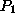and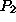. Production of these products is supported by two workstations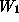and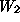, with each station visited by both product types. If workstationis dedicated completely to the production of product type, it can process 40 units per day, while if it is dedicated to the production of product, it can process 60 units per day. Similarly, workstationcan produce daily 50 units of productand 50 units of product, assuming that it is dedicated completely to the production of the corresponding product. If the company's profit by disposing one unit of productis \$200 and that of disposing one unit ofis \$400, and assumning that the company can dispose its entire production, how many units of each product should the company produce on a daily basis to maximize its profit?

Solution: First notice that this problem is an optimization problem. Our objective is to maximize the company's profit, which under the problem assumptions, is equivalent to maximizing the company's daily profit. Furthermore, we are going to maximize the company profit by adjusting the levels of the daily production for the two itemsand. Therefore, these daily production levels are the control/decision factors, the values of which we are called to determine. In the analytical formulation of the problem, the role of these factors is captured by modeling them as the problem decision variables:

•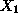:= number of units of productto be produced daily
•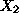:= number of units of productto be produced daily
In the light of the above discussion, the problem objective can be expressed analytically as: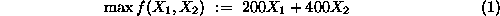Equation 1 will be called the objective function of the problem, and the coefficients 200 and 400 which multiply the decision variables in it, will be called the objective function coefficients.

Furthermore, any decision regarding the daily production levels for itemsandin order to be realizable in the company's operation context must observe the production capacity of the two worksationsand. Hence, our next step in the problem formulation seeks to introduce these technological constraints in it. Let's focus first on the constraint which expresses the finite production capacity of workstation. Regarding this constraint, we know that one day's work dedicated to the production of itemcan result in 40 units of that item, while the same period dedicated to the production of itemwill provide 60 units of it. Assuming that production of one unit of product type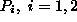, requires a constant amount of processing time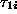at workstation, it follows that: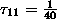and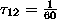. Under the further assumption that the combined production of both items has no side-effects, i.e., does not impose any additional requirements for production capacity of workstation(e.g., zero set-up times), the total capacity (in terms of time length) required for producingunits of productandunits of productis equal to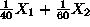. Hence, the technological constraint imposing the condition that our total daily processing requirements for workstationshould not exceed its production capacity, is analytically expressed by: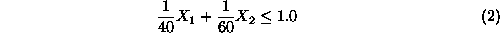Notice that in Equation 2 time is measured in days.

Following the same line of reasoning (and under similar assumptions), the constraint expressing the finite processing capacity of workstationis given by: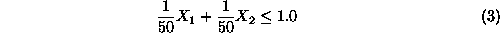Constraints 2 and 3 are known as the technological constraints of the problem. In particular, the coefficients of the variables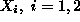in them,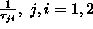, are known as the technological coefficients of the problem formulation, while the values on the right-hand-side of the two inequalities define the right-hand side (rhs) vector of the constraints.

Finally, to the above constraints we must add the requirement that any permissible value for variablesmust be nonnegative, i.e.,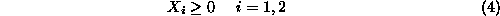since these values express production levels. These constraints are known as the variable sign restrictions.

Combining Equations 1 to 4, the analytical formulation of our problem is as follows: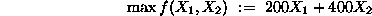s.t.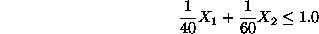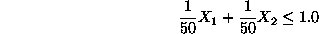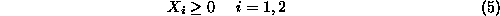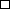Next: The general LP formulation Up: The LP formulation and Previous: The LP formulation and

UAL Data
Fri Jun 20 15:03:05 CDT 1997